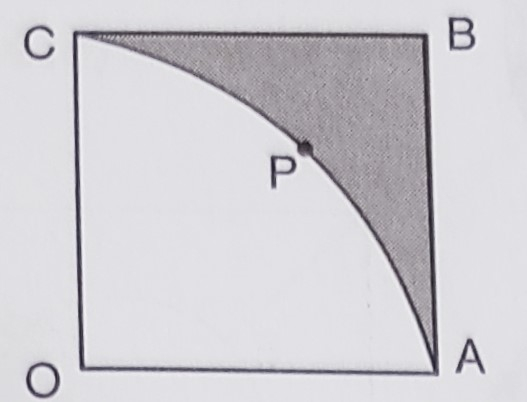"
">

# In the below figure, $O A B C$ is a square of side $7 \mathrm{~cm}$. If $O A P C$ is a quadrant of a circle with centre O, then find the area of the shaded region. (Use $\pi=22 / 7$ )"

Given:

$O A B C$ is a square of side $7 \mathrm{~cm}$.

$O A P C$ is a quadrant of a circle with centre O.

To do:

We have to find the area of the shaded region.

Solution:

Length of the side of the square $=7\ cm$

Area of the square $= (7)^2$

$= 49\ cm^2$

Radius of the quadrant $= 7\ cm$

Therefore,

Area of the quadrant $=\frac{1}{4} \pi r^{2}$

$=\frac{1}{4} \times \frac{22}{7} \times 7^2$

$=\frac{77}{2}$

$=38.5 \mathrm{~cm}^{2}$

Area of the shaded region $=$ Area of the square $-$ Area of the quadrant

$=49-38.5$

$=10.5 \mathrm{~cm}^{2}$

The area of the shaded region is $10.5\ cm^2$.

Updated on: 10-Oct-2022

43 Views